# Idempotent semi-ring

(diff) ← Older revision | Latest revision (diff) | Newer revision → (diff)

dioid

A semi-ring with idempotent addition. So, a setequipped with binary operations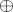(addition) and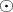(multiplication) and neutral elements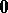andis called an idempotent semi-ring if the following basic properties are valid for all elements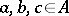:

i)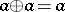(idempotent addition);

ii)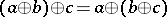;

iii)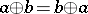;

iv)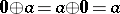;

v);

vi)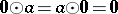;

vii)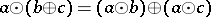;

viii)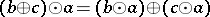.

An idempotent semi-ringis commutative if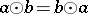for all. Different versions of this axiomatics are used, see e.g. [a1], [a2], [a3], [a4], [a5], [a6], [a7], [a8], [a9], [a10]. Idempotent semi-rings are often called dioids, see e.g. [a3], [a9]. The concept of an idempotent semi-ring is a basic concept in idempotent analysis. This concept has many applications in different optimization problems (including dynamic programming), computer science, automata and formal language theory, numerical methods, parallel programming, etc. (cf. also Idempotent algorithm and [a1], [a2], [a3], [a4], [a5], [a6], [a7], [a8], [a9], [a10], [a11]).

## Contents

### Example 1.

Let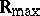be the set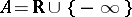(whereis the field of real numbers) equipped with the operations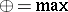and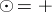(usual addition); set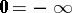,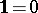. Similarly, let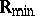be the set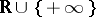equipped with the operations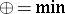and; in this case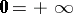and. It is easy to check thatandare (isomorphic) commutative idempotent semi-rings.

### Example 2.

Letbe the set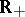of all non-negative real numbers endowed with the operationsand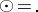(usual multiplication);,. This idempotent semi-ring is isomorphic to. The isomorphism is given by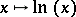.

### Example 3.with the operations,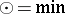and neutral elementsand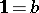(the cases,are possible).

### Example 4.

Let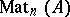be the set of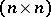-matrices with entries belonging to an idempotent semi-ring. Thenis a non-commutative idempotent semi-ring with respect to matrix addition and matrix multiplication.

The Boolean algebra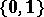is an example of a finite idempotent semi-ring. There are many other interesting examples of idempotent semi-rings, see e.g. [a1], [a2], [a3], [a4], [a5], [a6], [a7], [a8], [a9], [a10], [a11].

There is a natural partial order on any idempotent semi-ring (as well as on any idempotent semi-group; cf. also Idempotent semi-ring). By definition,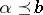if and only if. For this relation, reflexivity is equivalent to idempotency of the (generalized) addition, whereas transitivity, respectively anti-symmetry, follow from associativity, respectively commutativity, of this operation. On(and also on the semi-rings described in the Examples 2 and 3), this ordering relation coincides with the natural one; onit is the opposite of the natural ordering relation on the real axis. Every elementin an idempotent semi-ringis "non-negative" :. Indeed,. Similarly, for allone has, and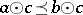if. Using this standard partial order it is possible to define in the usual way the notions of upper and lower bounds, bounded sets,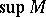(and) for upper- (lower-) bounded sets(respectively,), etc.

If the multiplication in a semi-ringis invertible on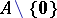, thenis called a semi-field. For example,is a semi-field. Idempotent semi-fields and semi-rings with idempotent multiplication are especially interesting.

How to Cite This Entry:
Idempotent semi-ring. Encyclopedia of Mathematics. URL: http://encyclopediaofmath.org/index.php?title=Idempotent_semi-ring&oldid=16503
This article was adapted from an original article by G.L. Litvinov (originator), which appeared in Encyclopedia of Mathematics - ISBN 1402006098. See original article